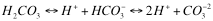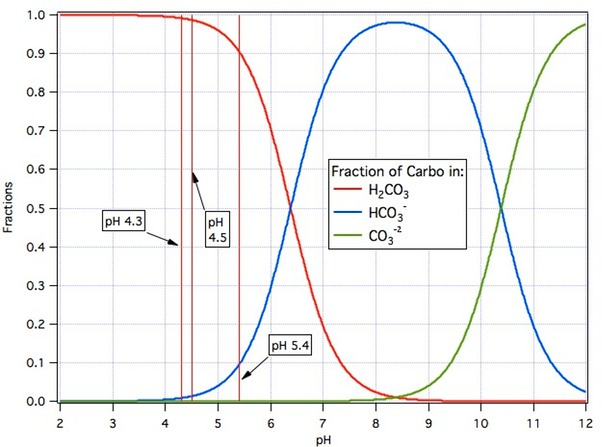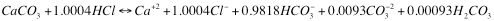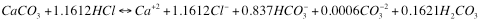# pH a different perspective (AJ deLange)

`I would like to thank AJ deLange for permission to re-post his pages.`

A Different Perspective on pH

Advances in technology have resulted in pH meters with performance high enough and prices low enough that it is possible for a home brewer on almost any budget to afford one. Accordingly, a lot of questions about their use arise. Here we have two pages, the first dedicated to the practical aspects of calibration and measurement (here) and the second to the mathematical details of the calibration algorithm (here). The former was originally posted on the Homebrew Talk site and readers may wish to visit that site in order to avail themselves of related questions and answers on this subject which appear there from time to time. The result of all this that brewers are now striving to hit proper pH at several points in their brewing process without perhaps fully understanding what pH is or why it should be controlled to a particular value. We will argue here that this is perfectly proper on the basis that brewers have for years been controlling temperature throughout the brewing process without fully understanding what temperature is though they generally do know why it must be controlled. You are thinking “Of course I know what temperature is!” but I suspect that few of you actually do. The goal of this page is to draw comparisons between temperature and pH to get you to the point where you are as comfortable with pH as you are with temperature. We’ll do this by mystifying temperature a bit while simultaneously demystifying (we hope) pH.

Temperature – What is it?

The obvious answer is that it is a measure of how hot something is but what does that really mean? We usually don’t bother to ask or answer such questions because our interest in temperature is entirely practical. If it is 35 degrees out on a given day we dress very differently than if it is 5 without giving it a second thought beyond whether we are comfortable or not. In this regard temperature and pH are different. We are constantly aware of temperature not only with regard to our comfort at a particular moment but with regard to things like cooking where we know chicken should be done at 180 degrees and salmon at 230  and brewing where we know that sachharification at 63C will result in a more fermentable beer than saccharification at 69C But how often do we think about pH in our daily lives? Whenever we brew or poach an egg or make a Hollandaise sauce or add more sugar to lemonade we are making from fresh lemons or when we add pickling lime to a batch of dills we are responding to pH but most are probably not aware of that fact.

If we do think about temperature beyond what numbers imply comfort and what numbers produce good beer it is in terms of energy. We know that to increase the temperature of a system we need to apply heat and that heat is a form of energy (or so we think – heat is actually the transfer of energy by certain means). You can skip the next three paragraphs if you don’t care that much about what temperature actually is.

We can get an idea as to what temperature might be by looking at the ideal gas law: PV=nRT in which P is the pressure, V the volume, n the number of atoms of the gas in the volume, R a constant and T the temperature. Rearranging this to P=nRT/V we can then look at the pressure on the walls of the container in which this gas is held. We assume that the container is a perfect insulator. This means that no energy can escape it which implies that no heat can pass through it. This, in turn, implies that if an atom of the gas strikes one of the walls that none of its energy will be imparted to the atoms or molecules in the wall or, put another way, that the collision is perfectly elastic so that the energy of motion of the particle before and after the collision will be the same. This means that if a particle strikes the surface with velocity normal to it vn and vp parallel to it it must leave with normal velocity -vn and parallel velocity vp. For this to be the case the wall must impart an impulse (integral of force the time during which the force acts) = 2mvn where m is the mass of the particle. vn is a function of the magnitude of velocity, v, of the particle given by the magnitude of v times the cosine of the angle between the velocity and the normal. The impotant concept is that it is proportional to the speed of the particle.

The volume, V, of the container is proportional to the cube of some linear measurement made upon it such as the radius of a sphere or the height of a cylinder. The shape doesn’t change this cubed relationship: only the proportionality constant changes as the shape changes. Calling this linear dimension l it should be clear that when a particle strikes the wall of the container, and rebounds it will strike another wall some time later and that that time will be proportional to l and inversley proportional to the speed of the particle i.e. to l/v and that, therefore, the rate at which collisions occur must be proportional to nv/l and the total force exerted by the container walls must be proportional to the average impulse and the number of collisions per unit time i.e proportional to 2mv2/l. For every reaction there is an equal and opposite reaction so that there must be force on the walls equal to the force exerted by the walls. Pressure is the force per unit area and the area of the walls is proportional to l2 so the pressure on the walls of the container would be proportional to 2nmv2/l3 and, as volume is proportional to the cube of the linear dimension, also to 2nmv2/V. Comparing this to P=nRT/V we see thatT must be proportional to mv2.

We have been very cursory here in using the same symbol, v, for velocity and speed and have glossed over the details of the averaging which is implied. In the final form, mv2, v2 is the average squared speed (magnitude of velocity) of the particles and is thus twice the average kinetic energy of the particles and this is the result we sought: the temperature of an ideal gas is a measure of the average kinetic energy of its particles. In a real gas or in a solid or liquid things become much more complicated and we must include the effects of particle/particle collisions as well as collisions with the walls and in diatomic or molecular gasses vibrational and rotational kinetic energy as well as translational but the result is the same: temperature is proportional to the average kinetic enrgy of the particles of the system. This definition is of little value to us when making beer. Another definition of temperature is that it is the first derivative of Gibbs energy with respect to entropy. I expect this definition is of even less use.

pH – What is it?

For the purposes of this discussion it is another parameter, like temperature, which you need to control to make good beer. We could stop right here but whereas readers are probably thoroughly comfortable with temperature, even though most probably don’t know what it really is, they are probably not so comfortable with pH as they don’t experience it in the same way as temperature i.e. they probably don’t have a ‘hands on’ feel for it the way they do with temperature. But let’s think about this for a minute. There is a physical sensation associated with pH and that is sour taste for low pH value and bitter taste for high. Something which is at high temperature feels hot. Something at low pH tastes sour. If you touch something that is at a very high temperature or at a very low temperature tissue damage will result. The same will happen if you expose yourself to excessively high or low pH. If you try to cook and egg at too high or low a temperaure range you will not get a properly cooked egg but, as many may know, there is a reason why cooks add vinegar to the water in which they poach eggs or to hollandaise sauces and that is to control the pH. You already know, or are learning, that it is not sufficient to control mash temperature for best results. pH must be controlled too.

Thus we see that there are parallels between pH and tempertaure in the sense that we can physically feel both, that we can hurt ourselves if exposed to materials outside a particular range of either and that we must control both in activities like cooking and brewing (and many others as well). At the completion of our discussion of pH we will see that for the brewer pH and temperature have one major thing in common: they are both things we can control and we do so because both effect the shape of protein molecules.

As we did above for temperature we will now discuss in more technical terms what pH is. In this case we’ll start with the most precise definition and work that back to more understandable terms. pH is a measure of the activity of hydrogen ions. The formal definition comes from the International Union of Pure and Applied Chemists (IUPAC), an international standards body concerned with matters chemical. The activity of a species is, as the name suggests, representative of its ability to participate in chemical reactions. The IUPAC definition of pH indicates that it is minus the logarithm to the base 10 of the molality scale concentration of hydrgen ions multiplied by an activity coefficient. When the hydrogen ion concentration is weak (pH between 4 and 10) and the solutions dilute the activity coefficient is close to 1 and the molality concentration (moles of ion per kilogram of solvent) and molar concentration (moles per liter of solution) will be very close to the same. Finally as the molecular weight of hydrogen ions is 1 gram per mole a concentration of 1 mole/kg corresponds to 1 gram/kg or approximately 1 gram/liter. Also as the hydrogen ion carries a unit of electronic charge 1 mole/kg corresponds to 1 eqivalent per kg or 1 equivalent per liter. The result of all this is that while pH is in fact precisely defined as the negative logarithm of the activity on the molal scale you can think of it in terms of the negative logarithm of the concentration expressed as grams/L. As an example,1 L of deionized water to which has been added 1 millimole (36.45 mg) of hydrochloric acid would have a hydrogen ion concentration close to 1 mmol/L (0.001mol/L) which is very close to 0.001 mol/kg water because the solution contains almost 100% water. As the solution is dilute (36 mg is only 0.004% of a kg) the activity coefficient is nearly 1 and the activity of hydrogen would be very close to 0.001 mol/kg (the activity coefficient is dimensionless) and the pH thus -log(.001) = 3. If we added 3.645 mg of hydrochlorc acid to 1L of water the concentration of hydrogen ions would be 0.0001 mol/kg and the pH 4. If we added 0.3645 mL of hydrochloric acid the hydogen ion concentration would be 0.0000101 mol/kg and the pH 4.9957 or, to two decimal places, 5.00. You are doubtless asking yourself ‘Where did that 0.0000001 mol/kg come from and why didn’t it appear in the pH 3 and 4 cases?’. The answer is that it comes from the water itself and that it does appear in the pH 3 and 4 cases but given that, even in the pH 5 case, it has no effect unless we try to measure pH to more than 2 decimal places, it has even less so at pH < 5 and we can ignore it.

Water is HOH or, if you prefer H20. A tiny fraction of the water molecues in any solution, separate into H+ and OH- ions. In pure water at 24.8°C that fraction is such that the concentration of hydrogen ions (equal to the concentration of hydroxyl ions) is 0.0000001 mol/kg = mol/L = gram/L. This lets us answer a second question: ‘What is the pH of pure water?’ and, as apparently, the hydrogen ion concentration is 0.0000001 in pure water, the answer is pH = 7. This is the case for pure water at 24.8°C. At room/laboratory temperature the fraction of dissociatin water molecules is a bit less and the pH thus rises to 7.085. Does this make sense in terms of our earlier discussion of temperature? At lower temperature the average water molecule has less kinnetic energy, is not moving as fast and is less likely to suffer a collision with another water molecule of sufficient violence to dislodge a proton. Does this work the other way too? Indeed it does. At saccharification temperature of 60 °C (140 °F) the pH of pure water is 6.51.

pH = – log(concentration) is the definition you will usually see in beginning level textbooks but it is rare that you will be interested in the number of mg of hydrogen ions in a liter of water or beer. What you will be interested in is what the implications of a particular concentration of hydrogen ions (i.e. a particular pH) are with respect to acids.

pH and Acids

The reason we must control pH is that by so doing we can regulate the degree to which acids are dissociated i.e. the extent to which they give up hydrogen ions (which are protons). As a protons are positively charged, each time an acid gives up a proton it becomes negatively charged by 1 electronic charge unit. The end result is that at higher pH acid residues are more negatively charged than at lower pH because they have lost more protons.

The following chemical equation shows electrically neutral carbonic acid at the left shedding one proton to become bicarbonate ion with unit negative charge and then the second to become carbonate ion with double negative charge.We asserted above that high pH is required for this to happen but looking at the equation one sees more and more protons released as we move to the right and more protons present implies lower pH. There is no paradox here. To establish high pH and thus move this reaction to the right we need to absorb the protons. A consequence of Le Chatelier’s Principal is that if we remove something (protons) from the right hand side of a chemical equation (which we do by adding a base – a substance that absorbs protons) the reaction will proceed to the right releasing more to make up for the ones we have removed. The converse is also true. If we supply protons (which we do by adding acid – a substance which supplies protons) then the reaction will proceed to the left (notice that the arrows are double headed meaning that the reaction can proceed in either direction) thus absorbing protons and attempting to re establish an equilibrium. For any particular combination of carbonic acid and other acids or bases added to a given volume of water there is one extent to which the reaction moves forward which results in equilibrium. In adding these other acids and bases we are shifting the pH. The ultimate outcome depends on  the pH we set as illustrated in the following graph.The term ‘carbo’ on the graph is my collective term for the sum of the molar amounts of carbonic acid, bicarbonate ion and carbonate ion. It is the total moles of carbon in those three species.Starting with a given amount of carbonic acid in water (CO2 bubbled through the water volume results in the production of carbonic acid and lowering of the the pH) the red curve shows the fraction of it that remains as carbonic acid as the pH raised by adding base. The blue curve shows the fraction that converts to bicarbonate ion and the green curve the fraction that converts to carbonate ion. Or we could interpret the curves as describing what happens as limestone (CaCO3) dissolves and acid is added moving the pH to the left. Or we could interpret it as telling us what happens if we add sodium bicarbonate to water (the pH goes to 8.3, about 1% of the bicarbonate become carbonic acid and about 1% becomes carbonate ion) as we add either acid or base to shift the pH away from 8.3.

By using the graph, or numbers derived from the same calculations used to prepare the graph, we can write balanced chemical equations covering the situation where, for example, we dissolve a unit of limestone with hydrochloric acid and adjust the pH, by controlling the total amount of hydrochloric acid we add, to a particular desired value. If that value is pH 8.71 the equation look like thisand, if it is pH 7.00 it looks like this:It should be intuitively pleasing that it takes more acid to reach the lower pH. Readers should also take note that while the total charges on ions are 0 in both cases there is more negative charge on carbo species in the higher pH case than in the lower one (where the remaining charge required for balance is carried by chloride ion).

For brewers limestone dissolved in water is the source of alkalinity and alkalinity is a brewers major water related concern. In nature the dissolution of limestone is aided by carbonic acid which comes from respiring bacteria resident in the soil. The deatails of how this takes place are at the heart of brewing water chemistry but they are intricate if not complex and we have lost many brewers by the wayside in trying to explain them over the years. They are all set out in this paper which was published in Cerevesia in 2004.

The three pH values marked on the graph are, respectively from the left, two common pH values used for the end point titration in alkalinity determination and a typical mash pH. In measuring alkalinity we add acid to a sample of the water in question until the end point pH is reached i.e. until, per the graph, nearly all the carbonate and bicarbonate have been converted to CO2. In adjusting mash water for proper mash pH we do essentially the same thing but don’t go so low in pH.

pH and Protein

We have shown how we can control the charge on acid anions by varying pH. A protein is made up of a chain of amino acids some of which have charged side groups which behave like acids or bases. By varing the pH we can control the extent to which these side groups lose or retain their protons and thus whether they assume negative 0 or positive charge in accordance with the pricipal that higher pH always implies less positive (more negative) charge. pH, therefore, gives us control over the charge distribution over the length of the protein molecule. As like charges repel one another and opposite attract the shape of the molecule depends on the charge distribution and we can, thus, vary the shape of a protein molecule by varying pH. The enzymes upon which we depend for catalysis of the multiple reactions which take place in all phases of the brewing process are simply proteins. Their abilities to act depend on their shapes and thus on the pH of the environment in which they operate. This is why we must have suitable pH in the mash tun and in the fermenter.

Summary

pH and temperature are both sensible physical quantities which we can measure:

High pH substances taste bitter. High temperature substances feel warm
Low pH substances taste sour. Low temperatire substances feel cool

pH and temperature are both physical quantities that we can control: